31 篇文章 46 订阅

[四足机器人]开环运动控制测试

# 第一章 序

①平衡性和动态运动能力:能够让机器人在任意地方、任何地形保持平衡，并实现自由活动，这意味着机器人的工作范围得到了有效扩展。

②对运动的控制能力:指机器人可以灵活地操控物体（如使用键盘和遥控器等），同时进行自由活动，这意味着机器人能够在移动的过程中轻松完成各项操作任务。

③移动感知能力:指机器人能够感知空间中物体的稳定存在，即便视线移向别处也能够避开障碍物，这意味着机器人能够绘制出周边环境中障碍物的位置图，从而在移动的过程中能够有效避开障碍物。

# 第二章 运动状态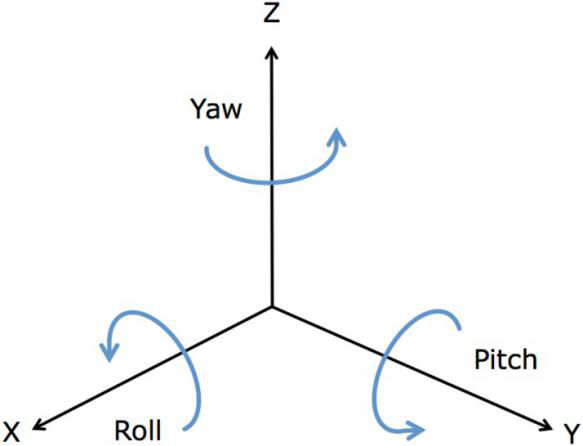## 运动控制

1、运动分解

①沿X轴的平移（Liner_X）
②沿Y轴的平移（Liner_Y）
③绕Z轴的旋转（Yaw）

2、运动叠加

①+②：平面内任意方向的斜向直线运动
①+③：指定半径的转向运动

# 第三章 步态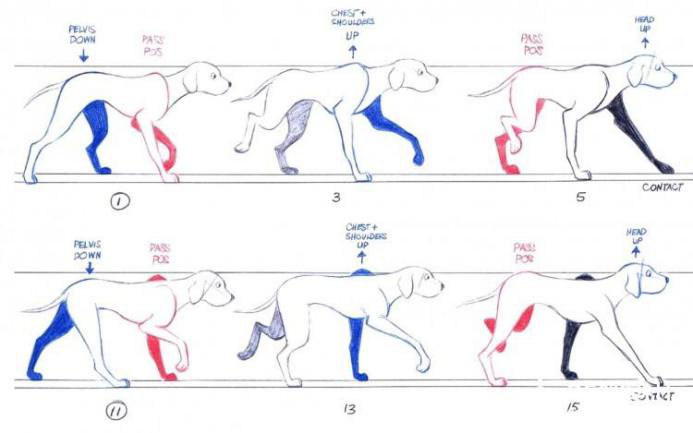①步态周期T：一个完整运动循环所用的时间；
②步长S：一个步态周期内，支撑腿驱动躯体质心相对于地面移动的距离；
③抬腿高度h：一步内足端离地最大距离；
④相位差 φ i \varphi_i ：第i条腿着地时刻相对于参考腿的延时与周期的比；
⑤支撑（stance）：腿与地面接触支撑躯体并推动躯体前行的状态；
⑥摆动（swing）：腿抬起在空中摆动的状态；
⑦负载因子 β \beta ：处在支撑相的腿撑在地面的时间占整个运动周期的比例。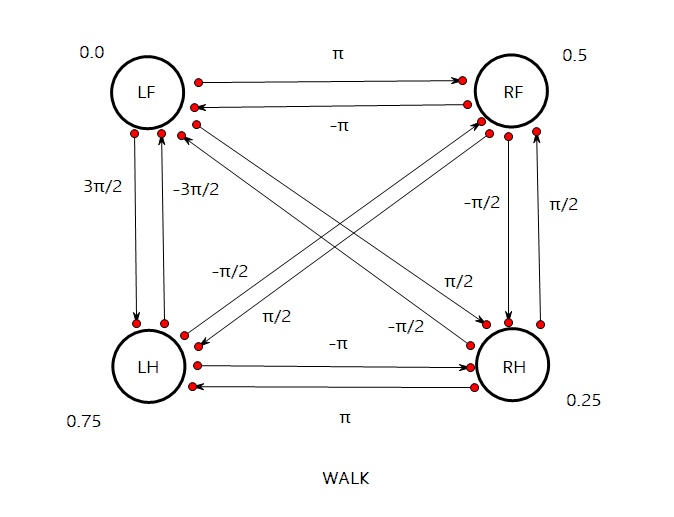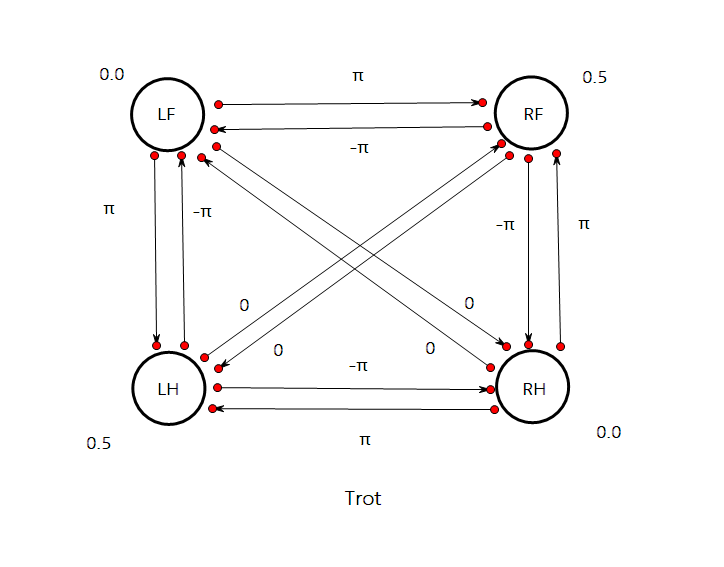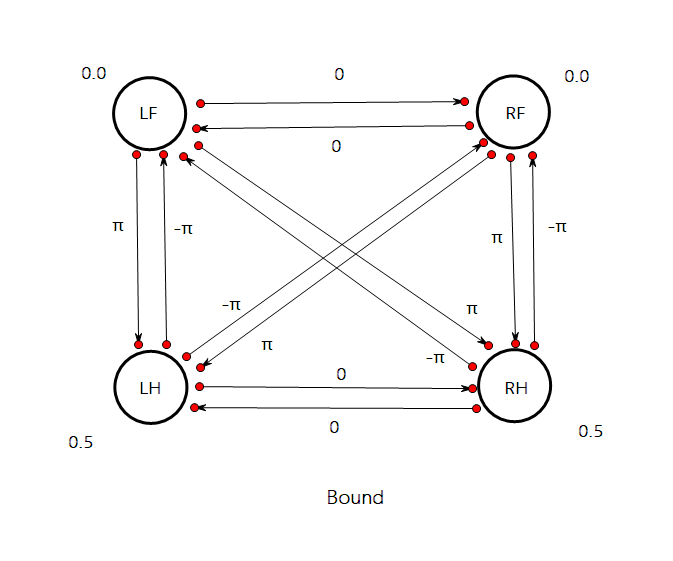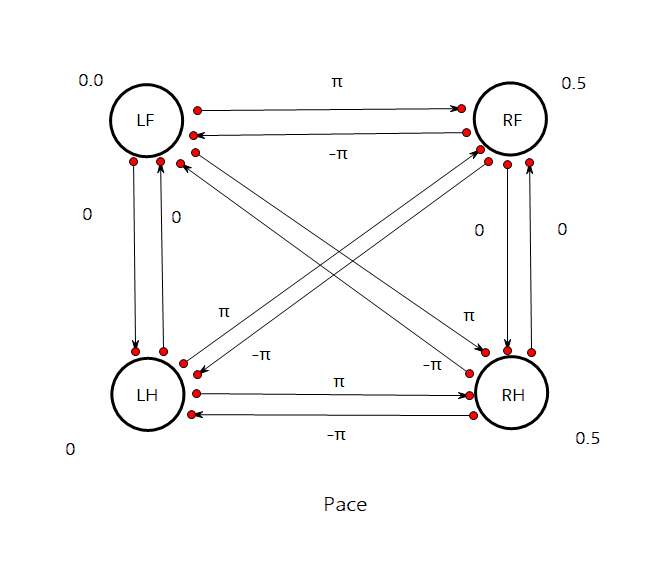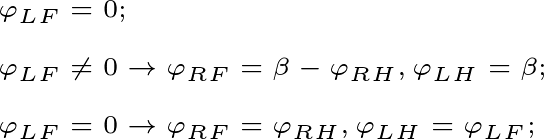# 第四章 CPG控制网络

## CPG模型分类

①基于神经元的模型：Matsuoka神经元震荡模型、Kimura模型等，该类模型生物学意义明确，但参数较多，动态特性分析比较复杂。

②基于非线性振荡器的模型：Kuramoto相位振荡器、Hopf谐波振荡器等，该类模型参数较少，模型比较成熟。

## 基于HOPF振荡器的CPG单元模型

1、HOPF振荡器

HOPF振荡器在状态空间中存在一个稳定的极限环，即对于任意非零初始值，均能使得振荡器产生相同形状的周期性振荡信号，其数学模型如下：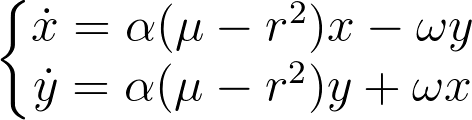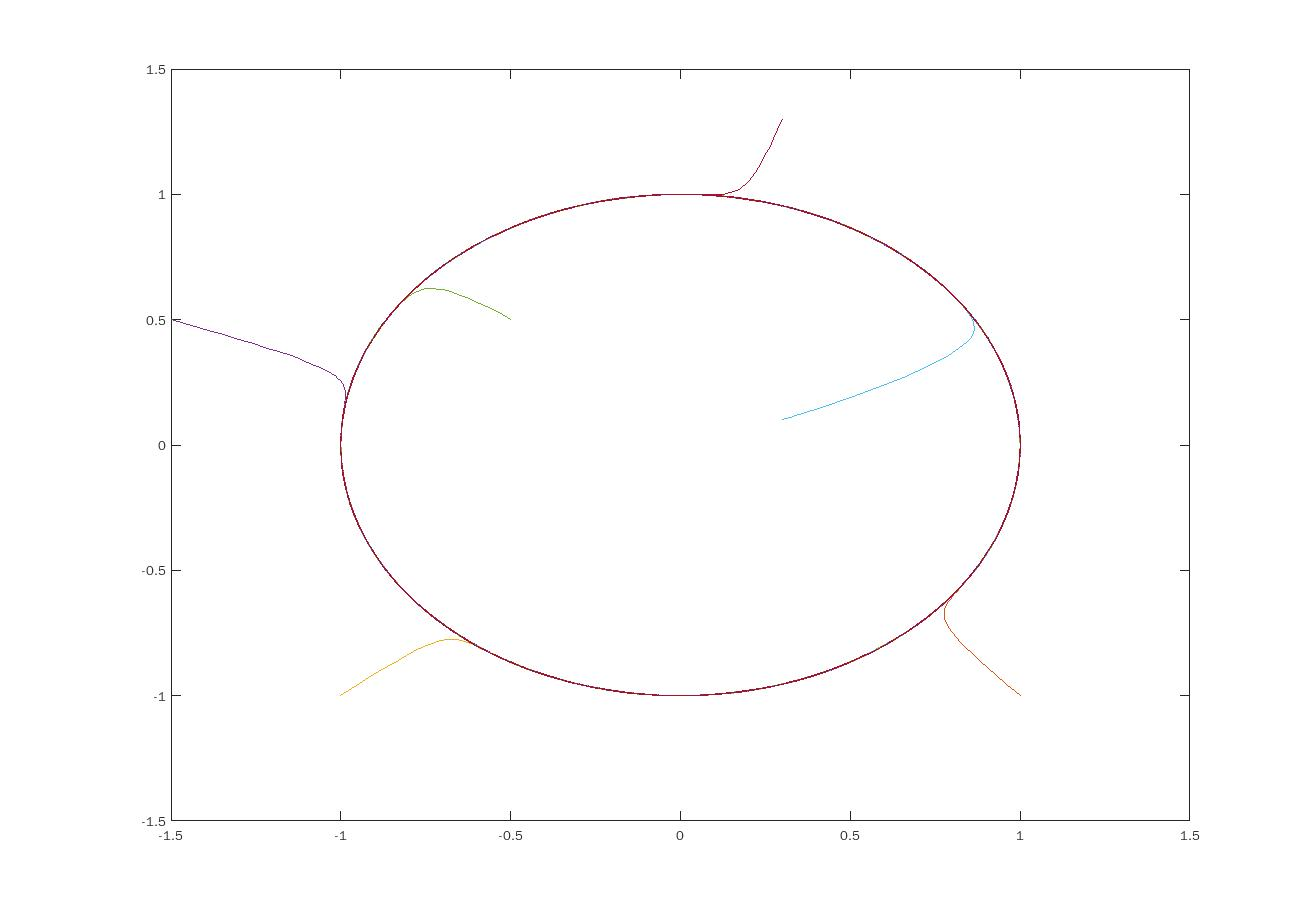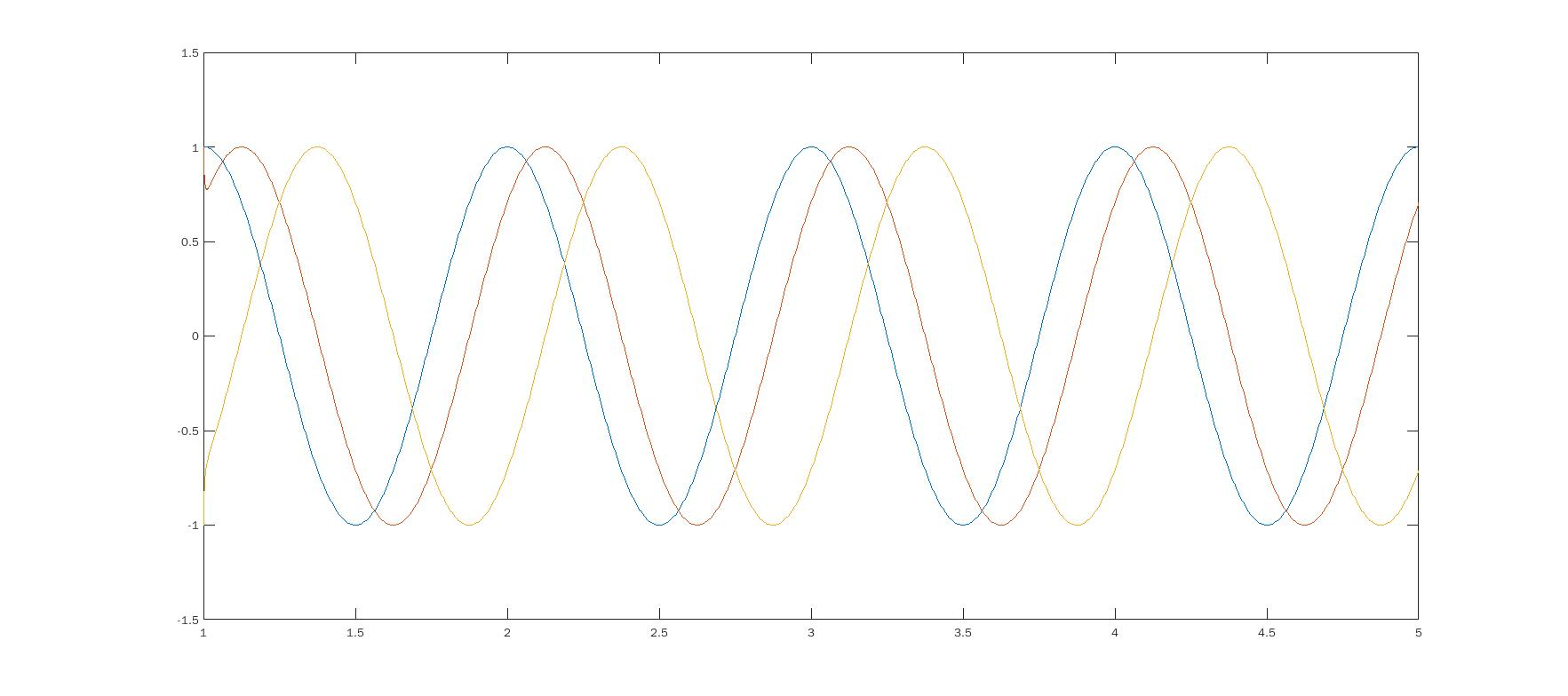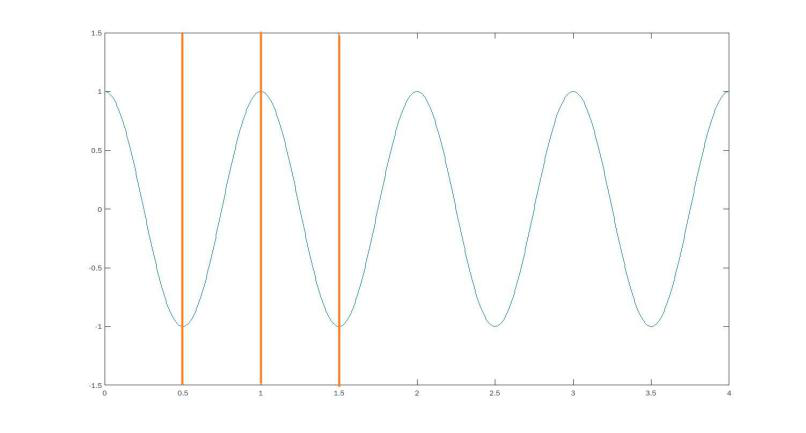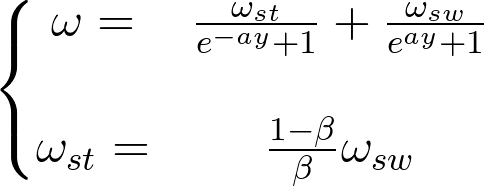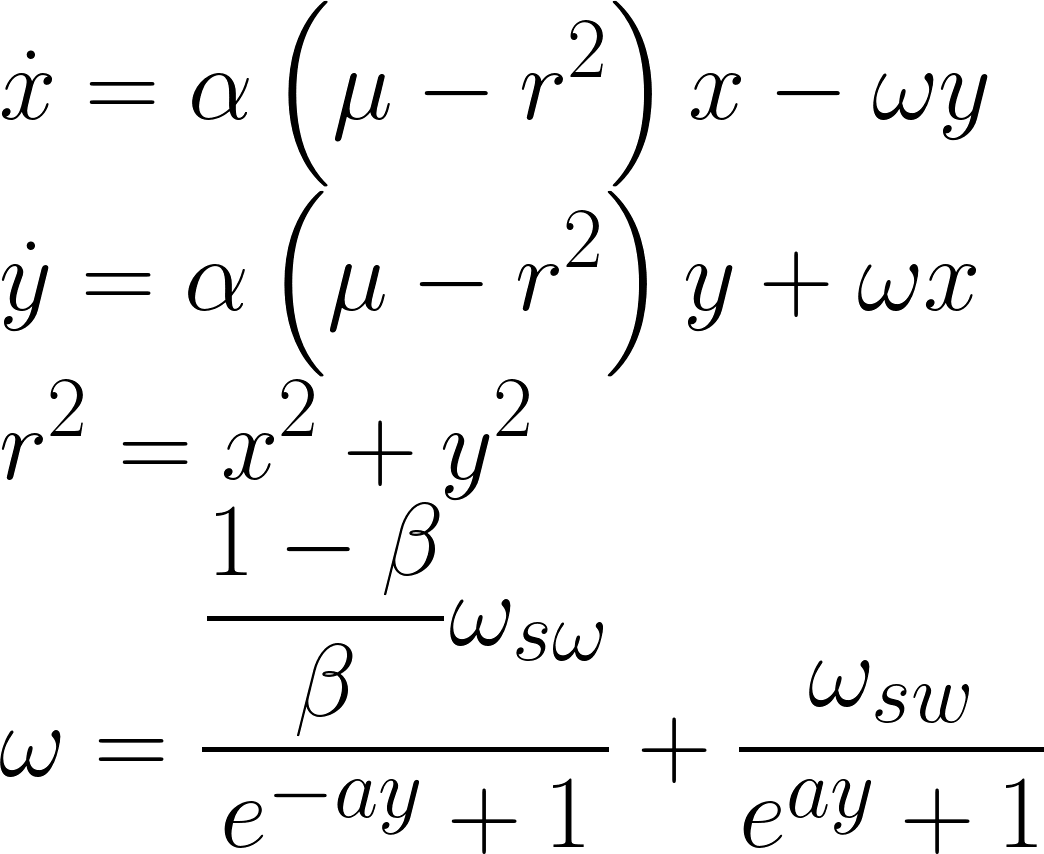α用于控制振荡器收敛到极限环的速度；
a决定了ω在 ω s t \omega _{st} ω s w \omega_{sw} 之间的变化速度；
μ决定振荡器的幅值，关系式为 A = μ A=\sqrt{\mu}
β为负载因子（范围0-1）；
ω s w \omega_{sw} 表示摆动相频率；
ω s t \omega_{st} 表示支撑相频率，且 ω s t = 1 − β β ω s w \omega _{st}= \frac{1-\beta}{\beta}\omega_{sw}

2、动态特性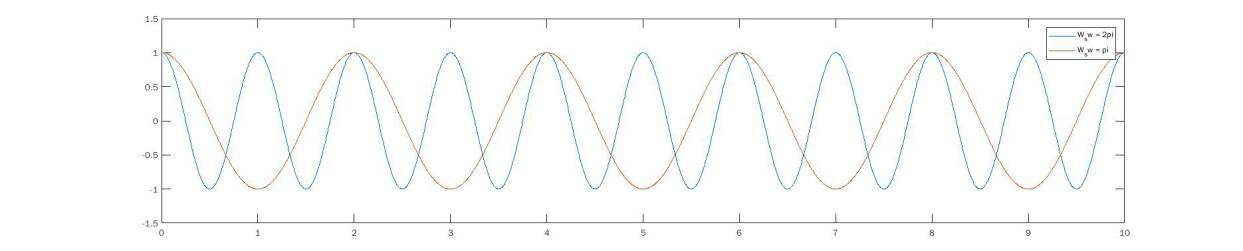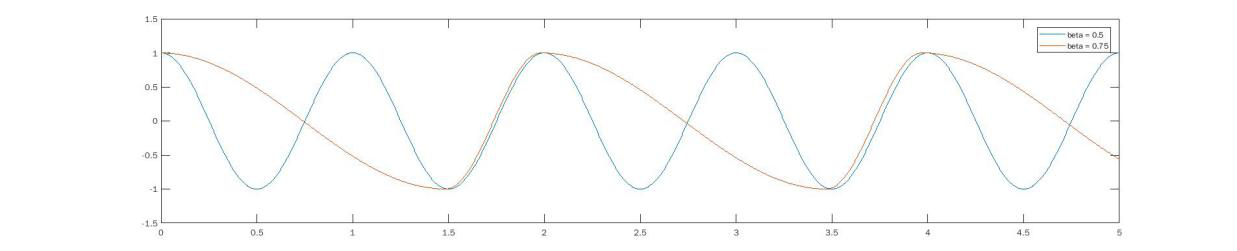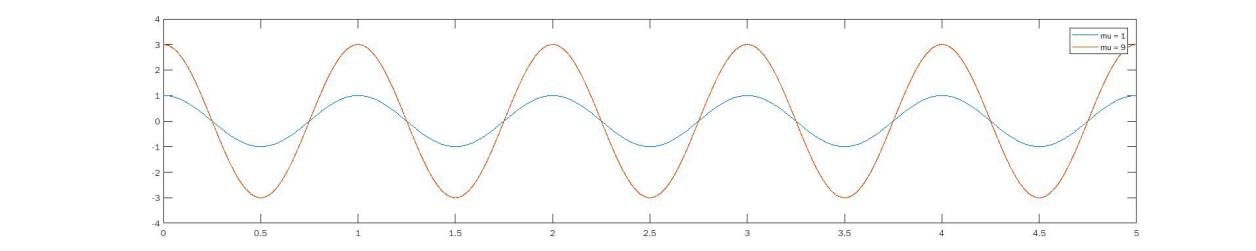μ \mu ：控制输出信号的幅值 A = μ A=\sqrt{\mu} ;
ω s w \omega_{sw} ：控制调节输出信号的周期;
β \beta ：控制输出信号上升/下降沿所占时间比例。

## CPG网络控制模型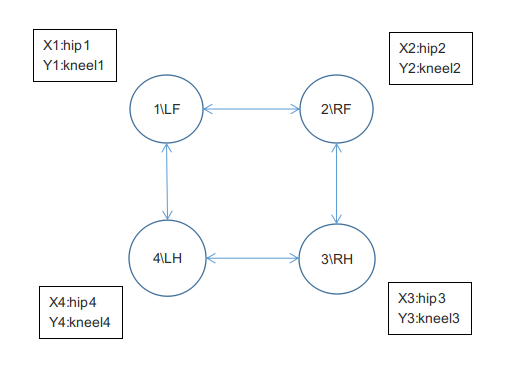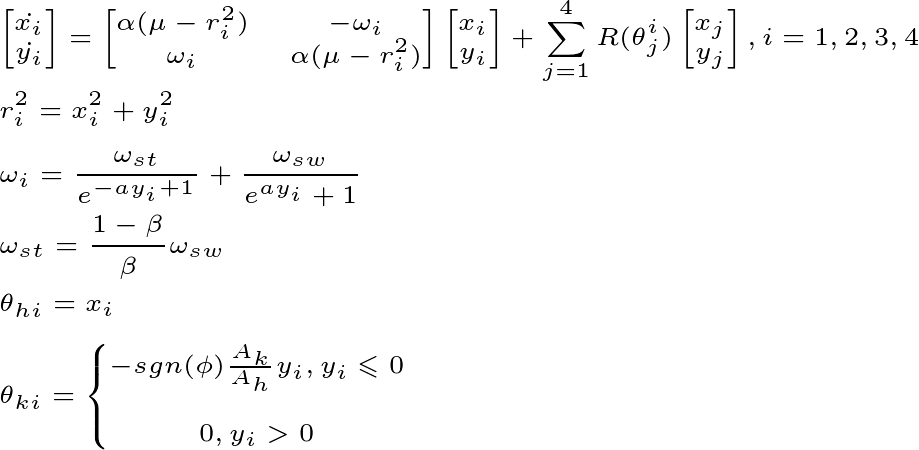A h , A k A_h,A_k 分别为髋关节，膝关节幅值， ϕ \phi 为关节标志，该项与硬件结构配置有关，我们的机器人采用前肘后膝式。即LF_k, RF_k为1， LH_k, RH_k为-1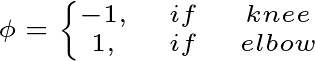R ( θ j i ) R(\theta^i _j) 控制各腿控制信号的耦合关系,且 θ j i = 2 π ( φ i − φ j ) \theta^i_j = 2\pi (\varphi_i-\varphi_j) φ i \varphi_i 为第i个振荡器的相位（相位图请看第三章：步态）,其数学表达式如下: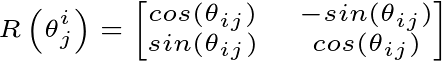③为了更加直观，我们把耦合项展开成以下矩阵形式（一个8*4矩阵，按行求和）: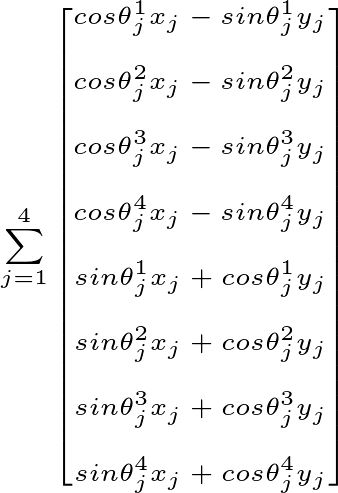④同样我们可以把数学模型写成更加直观的微分方程：

d x i d t = α ( μ − r i 2 ) x i 2 − ω i y i + ∑ j = 1 4 ( cos ⁡ θ j i x j − sin ⁡ θ j i y j ) \frac{dx_i}{dt} = \alpha(\mu-r_i^2)x_i^2 - \omega_iy_i+ \sum_{j=1}^{4}(\cos\theta^i_jx_j-\sin\theta_j^iy_j)

d y i d t = α ( μ − r i 2 ) y i 2 + ω i x i + ∑ j = 1 4 ( sin ⁡ θ j i x j + cos ⁡ θ j i y j ) \frac{dy_i}{dt} = \alpha(\mu-r_i^2)y_i^2 + \omega_ix_i+ \sum_{j=1}^{4}(\sin \theta^i_jx_j+\cos \theta_j^iy_j)

θ h i , θ k i \theta_{hi},\theta_{ki} 分别为第i条腿的髋关节，膝关节的控制信号，是一条关于时间的函数。

# Tips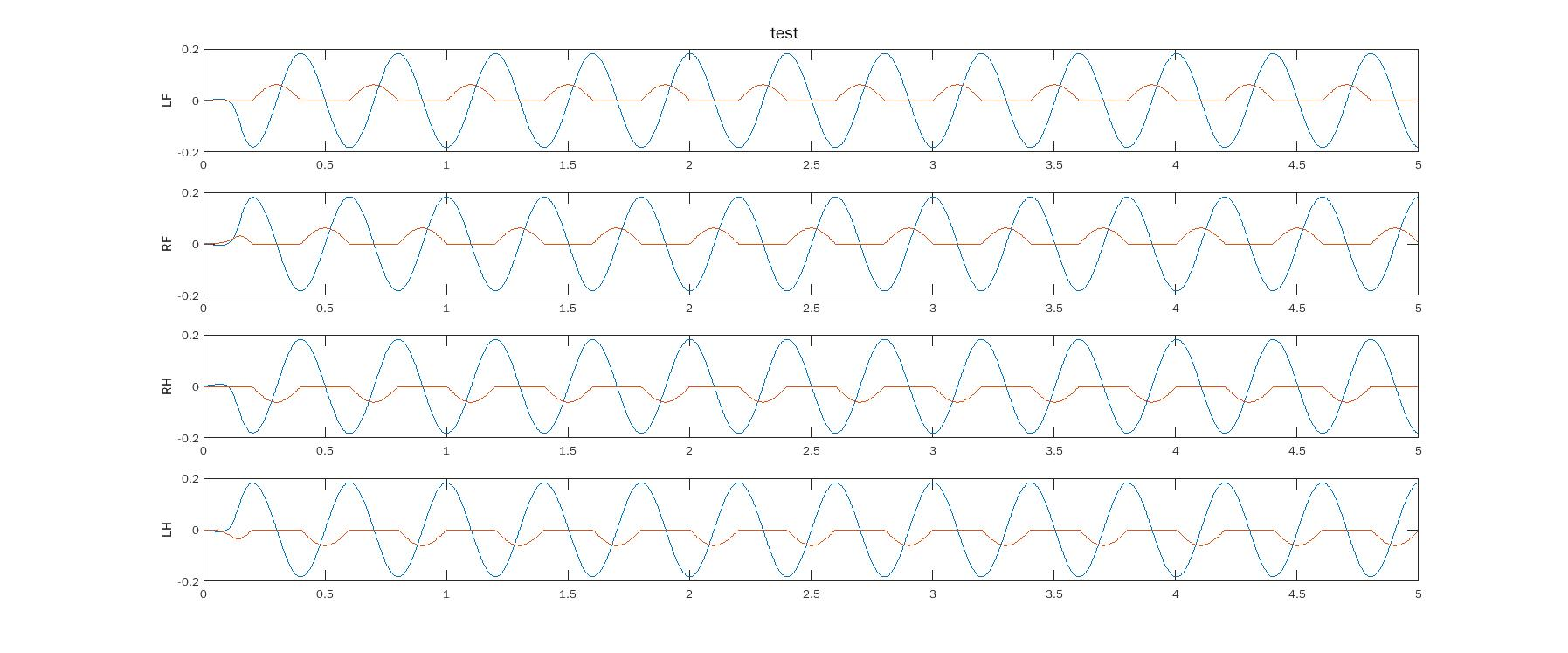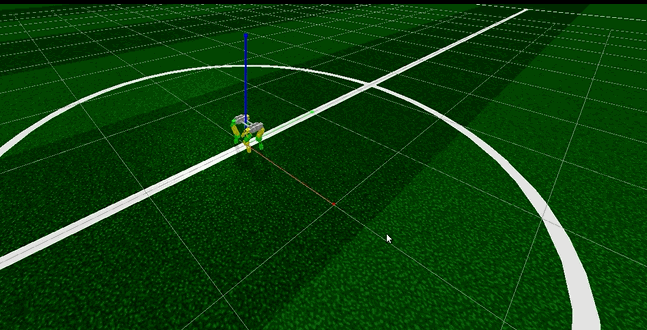# 参考文献

.罗庆生, 罗霄著. 仿生四足机器人技术[M]. 北京:北京理工大学, 2016. 15-30

韩宝玲, 王秋丽, 罗庆生. 六足仿生步行机器人足端工作空间和灵活度研究[J]. 机械设计与研究, 2006,22(4): 11-12

常青, 韩宝玲, 罗庆生. 四足机器人转向与斜向运动规划理论及方法[J]. 北京理工大学学报, 2015, 35(5): 1-2

施宏阳. 基于WCPG的步态生成与运动控制方法[D]. *:华中科技大学, 2015. 15-18

高翔. 视觉SLAM十四讲[M]. *:电子工业出版社, 201708-0310-311万+
08-25453
04-19759
07-304141
09-242078
08-205926
08-18757
10-17493
11-21738
03-012841
11-13
12-102万+
08-091万+
04-144064点击重新获取扫码支付余额充值